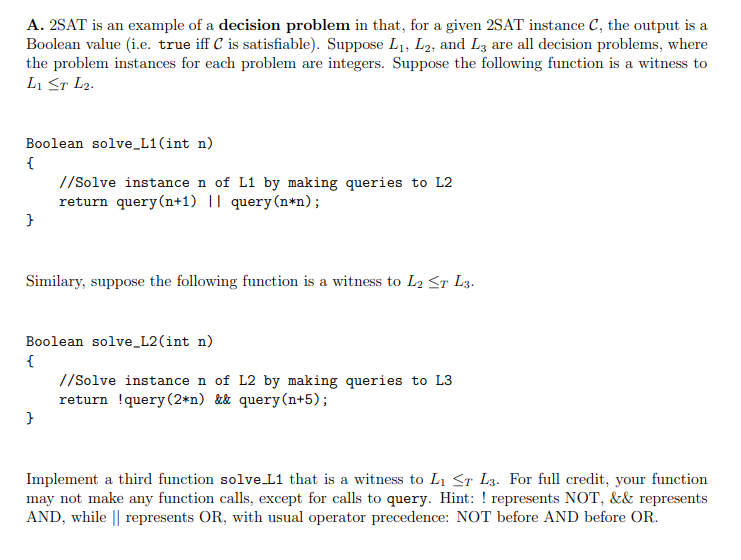# (Solved) : 2sat Example Decision Problem Given 2sat Instance C Output Boolean Value E True Iff C Sat Q43029985(1) . . .

\$9.00A. 2SAT is an example of a decision problem in that, for a given 2SAT instance C, the output is a Boolean value (i.e. true iff C is satisfiable). Suppose L1, L2, and L3 are all decision problems, where the problem instances for each problem are integers. Suppose the following function is a witness to L1 ST L2 Boolean solve_L1(int n) //Solve instance n of L1 by making queries to L2 return query(n+1) | query(n*n); Similary, suppose the following function is a witness to L2 <r L3. Boolean solve_L2(int n) //Solve instance n of L2 by making queries to L3 return !query (2*n) &k query(n+5); Implement a third function solve L1 that is a witness to L1 St L3. For full credit, your function may not make any function calls, except for calls to query. Hint: ! represents NOT, && represents AND, while represents OR, with usual operator precedence: NOT before AND before OR Show transcribed image text A. 2SAT is an example of a decision problem in that, for a given 2SAT instance C, the output is a Boolean value (i.e. true iff C is satisfiable). Suppose L1, L2, and L3 are all decision problems, where the problem instances for each problem are integers. Suppose the following function is a witness to L1 ST L2 Boolean solve_L1(int n) //Solve instance n of L1 by making queries to L2 return query(n+1) | query(n*n); Similary, suppose the following function is a witness to L2